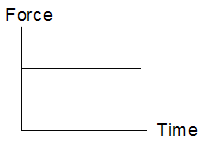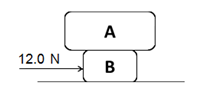Chapter 3 Forces Part 2 - Newton's Second Law
• recall and apply the relationship resultant force = mass x acceleration to new situations or to solve related problems

Newton's second law of motion pertains to the behavior of objects for which all existing forces are not balanced. The second law states that the acceleration of an object is dependent upon two variables - the net force acting upon the object and the mass of the object
Name *
Class *
Index Number *
What expression can be used to calculate force? *
1 point
When a force is applied to a body, several effects are possible. Which effect could not occur? *
1 point
A steadily increasing force applied to a moving motor car could result in a change in each of the following except the car's *
1 point
A known force is applied to an object on a horizontal, frictionless surface. What property of the object must be known in order to calculate its acceleration? *
1 point
The unit of force is the (1). An object subjected to (2) forces will accelerate. Acceleration is the change of (3). Which words correctly represent (1), (2) and (3)? *
1 point
The force-time graph shows how net force acting on an object varies with time. The object is initially at rest. Which of the following shows the velocity-time graph of the object? *
1 pointA 4.0 kg box A is stacked on top of a 2.0 kg box B. A force of 12.0 N is applied on box B. Assume that the surface that the boxes moved on is frictionless and that boxes A and B are glued together. What will happen to the motion of boxes? *
1 point